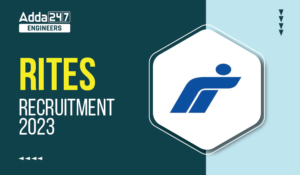Engineering Jobs   »   Mechincal Engineering quizs   »   Mechanical Engineering Quiz

# UPSSSC JE’21 ME: Daily Practices Quiz. 13-Sep-2021

Quiz: Mechanical Engineering
Exam: UPSSSC
Topic: Miscellaneous

Each question carries 1 mark
Negative marking: 1/4 mark
Time: 8 Minutes

Q1. The damping ratio in case of critical damping is…………………….
(a) Equal to one
(b) Equal to zero
(c) Less than one
(d) Greater than one

Q2. A differential gear in an automobile is a
(a) simple gear train
(b) epicyclic gear train
(c) compound gear train
(d) speed reducer

Q3. The thrust approaches to ……… when the propulsive efficiency of a turbo-jet aircraft approaches 100%
(a) Maximum
(b) 50% of the maximum
(c) 25% of the maximum
(d) Zero

Q4. In powder metallurgy, the operation carried out to improve the bearing property of a bush is called
(a) Infiltration
(b) Impregnation
(c) Plating
(d) Heat treatment

Q5. When an ordering cost is increased to 4 times, the EOQ will be increased to
(a) 2 times
(b) 4 times
(c) 8 times
(d) 16 times

Q6. The shearing area of a key of length ‘l’, breadth ‘b’ and depth ‘d’ is equal to
(a) l×d
(b) b×d
(c) l × b
(d) l×d/2

Solutions
S1. Ans. (a)
Sol Damping factor is the ratio of damping coefficient (c) and critical damping coefficient (Cc)
Damping factor, ς=C/c_c
ς = 1, critically damped system
ς > 1, over damped system

S2. Ans. (b)
Sol. differential gear mechanism works on the principle of epicycle gear train.When the car is on a straight road, the ring gear, differential cage, differential pinion gear and two differential side gears all tum as a unit. The two differential pinion gears do not rotate on the pinion shaft; therefore, they exert equal force on the two differential side gears. As a result, the side gears turn at same speed as the ring gear, which causes both the drive wheels to turn at same speed. However, when the car begins to round a curve, the differential pinion gears rotate on the pinion shaft. This permits the outer wheel to turn faster than the inner wheel.
S3. Ans. (d)
Sol.
Propulsive efficiency is maximum when jet velocity (C_j )= forward velocity of aircraft (C_a)
Now thrust power = (C_j-C_a ) C_a=0
i.e. at maximum efficiency thrust power is zero.
S4. Ans. (a)
Sol.
Q=kA(T_1-T_2 )/l
=(0.5×2×50)/0.25=200 W
S4. Ans.(b)
Sol. Bearings…………..Impregnation
S5. Ans.(a)
Sol. When an ordering cost is increased to 4 times, the EOQ will be increased to 2 times.
S6. Ans. (c)
Sol. Shearing area of key = Length (l) × Breadth (b) = l × b

Sharing is caring!

•GATE Result 2023 Out, Download Result, C...
•GATE 2023 Admit Card Out, Download Link ...
•RRB ALP Previous Year Question Papers, D...
•DDA JE Result 2023 Out, Download Branch-...
•FCI JE Previous Year Papers, Download Ol...
•RITES Recruitment 2023 Out, Apply Online...
•AP EAMCET Results 2023, Check Result dat...
•IB JIO Salary 2023, Check Detailed Salar...